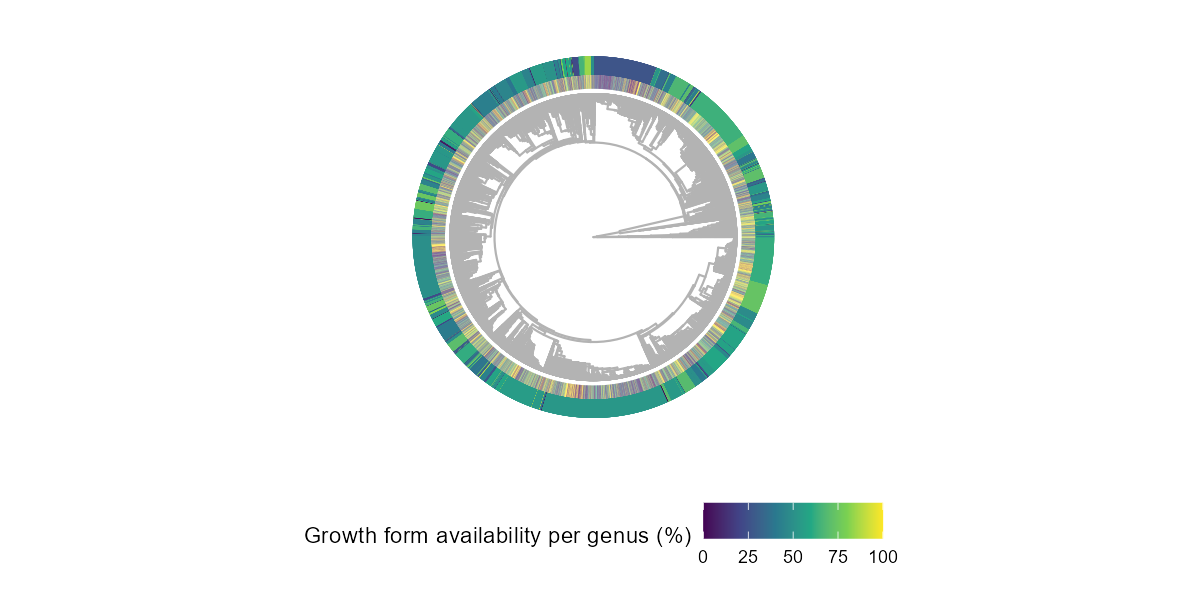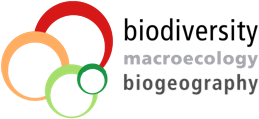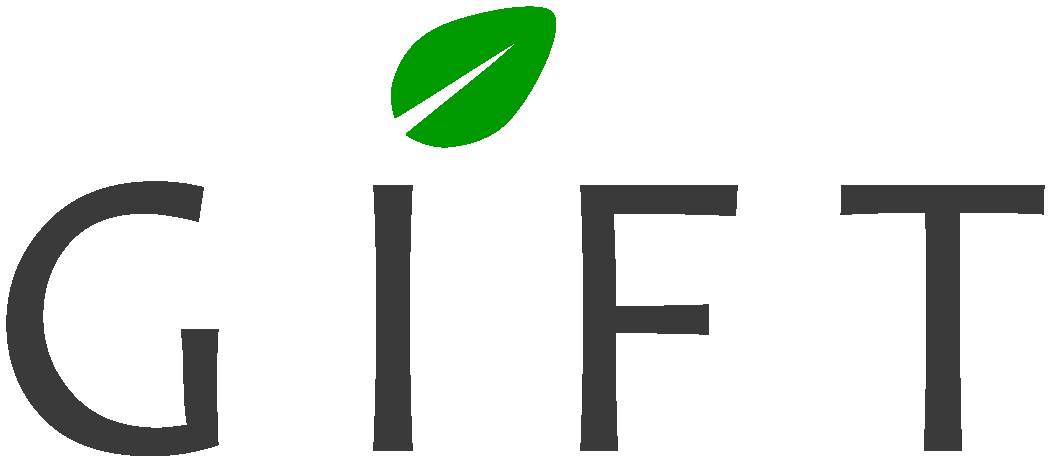The Global Inventory of Floras and Traits (GIFT) is a database of floras and plant checklists, distributed worldwide. It also includes trait and phylogenetic information. The GIFT R package grants an access to the GIFT database.
This vignette illustrates the most common uses of the package with detailed examples.

1. Retrieving plant checklists within a given area (example: Mediterranean)
2. Getting the distribution of a plant species
3. Retrieving trait information for a subset of plant species
4. Retrieving environmental information for a list of polygons/regions
5. Retrieving a plant phylogeny and plotting a trait coverage on it

The following R packages are required to build this vignette:

library("GIFT")
library("dplyr")
library("ggplot2")
library("sf")
library("rnaturalearth")
library("rnaturalearthdata")
library("tidyr")
library("patchwork")

Side-note
Some of the following queries may take a long time to complete Increasing the timeout makes it easier to complete larger downloads. This can be done as follows:

options(timeout = max(1000, getOption("timeout")))

## 1. Checklists for a region

### 1.1. Shapefile

Let’s assume we are interested in having a floristic knowledge of the western part of the Mediterranean basin. For this purpose, we can simply use a shape file of the region of interest and feed it to the GIFT_checklists() function.

We do provide a shape file of this region in the GIFT R package, which you can access using the data("western_mediterranean") command.

data("western_mediterranean")

world <- ne_coastline(scale = "medium", returnclass = "sf")
world_countries <- ne_countries(scale = "medium", returnclass = "sf")
# Fixing polygons crossing dateline
world <- st_wrap_dateline(world)
world_countries <- st_wrap_dateline(world_countries)

# Eckert IV projection
eckertIV <-
"+proj=eck4 +lon_0=0 +x_0=0 +y_0=0 +ellps=WGS84 +datum=WGS84 +units=m +no_defs"

ggplot(world) +
geom_sf(color = "gray50") +
geom_sf(data = western_mediterranean, fill = "darkblue", color = "black",
alpha = 0.5, size = 1) +
labs(title = "Western Mediterranean basin") +
lims(x = c(-20, 20), y = c(24, 48)) +
theme_void()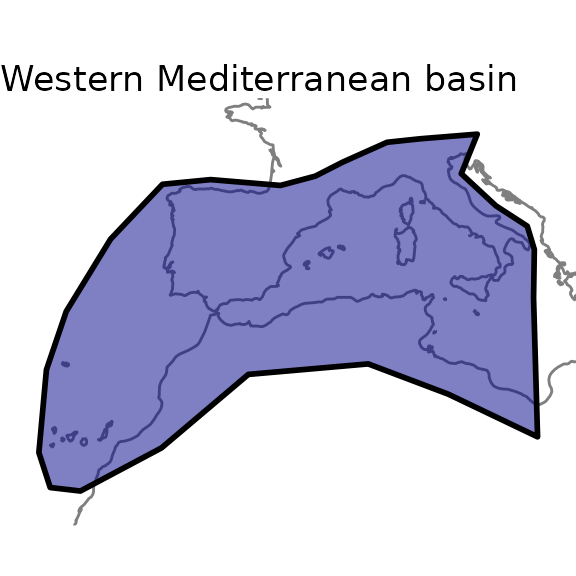Please note that shapes used in GIFT are unprojected (Geographic Coordinate System WGS84), and that all shapefiles provided should be in this CRS. You can check the coordinate reference system of a sf object by using sf::st_crs().

### 1.2. Main arguments

Now that we have a shape for the region of interest, let’s call GIFT_checklists(). This wrapper function has many arguments, which we detail in this subsection.
First, the taxonomic group of interest. We may be interested in a particular group of plants, say only Angiosperms. In this case, we would set the taxon_name argument like this taxon_name = "Angiospermae". If we are interested in a particular family of plants, let’s say orchids, then taxon_name = "Orchidaceae".
To see all the options for the taxon_name argument, you can run the GIFT_taxonomy() function and look at the taxon_name column of its output.
Along with this first argument comes complete_taxon. This argument, set to TRUE by default, determines whether only regions represented by checklists in GIFT that completely cover the taxon of interest should be retrieved. Figure 1 illustrates the principle.Figure 1. Principle of the complete_taxon argument

In Figure 1, we want to retrieve checklists of Angiosperms. In the first available region, region A, only one checklist is of interest. This checklist is then always retrieved. In region B, there is only one checklist of orchids, which is only a subset of Angiosperms. If complete_taxon is set to TRUE, then this checklist won’t be retrieved, otherwise yes. Finally, in region C, there is a checklist for vascular plants and one for orchids. In both cases, the checklist of vascular plants will be retrieved after filtering out the non-angiosperm species. The checklist of Orchids is also retrieved in both cases because it is not the only one available and because it can complete the floristic knowledge for Angiosperms in this region.

The following arguments of GIFT_checklists() refer to the floristic status of plant species. For example, we may be interested only in endemic or naturalized species. The default value is to get all native species.
Similarly, two arguments are needed in the function. First, floristic_group defines the group of interest. Second, complete_floristic indicates whether or not to retrieve incomplete regions with respect to the selected floristic group. The logic is detailed in Figure 2 and is similar to the complete_taxon argument shown aboveFigure 2. Principle of the complete_floristic argument

The next set of arguments relate to the spatial match between the desired area and the GIFT database.

The main argument in this regard, when providing a shapefile or a set of coordinates, is the overlap argument. This argument can take 4 options, each of which produces different result, as shown in Figure 3.In Figure3, the GIFT polygons shown in orange either intersect, fall inside or outside the provided shape file. The overlap argument below each GIFT polygon illustrates in which situation a given GIFT polygon will or will not be retrieved.

Another important spatial feature we provide is the possibility to remove overlapping polygons. In fact, for many regions of the world, there are several polygons in the GIFT database that cover them. If overlapping polygons are not an issue for your case study, you can simply set remove_overlap to FALSE (top right part of Figure 4). However, if you want to have only one polygon per region, you can set remove_overlap to TRUE. In this case, the GIFT_checklists() will either retrieve the smaller or the larger polygon. This depends on the values set for the area_threshold_mainland argument as shown in Figure 4.Figure 4. Removing overlapping polygons with remove_overlap argument

area_threshold_mainland takes a value in $$km^2$$. If the area of the smaller polygon is less than the threshold, then the larger overlapping polygon is retrieved (lower left part in Figure 4). If the smaller polygon exceeds the threshold, then it is retrieved (lower right part of Figure 4). There is a similar argument for islands, area_threshold_island, which is set to 0 $$km^2$$ by default. This way the smaller islands are always retrieved by default.

Note also that polygons are considered to overlap if they exceed a certain percentage of overlap. This percentage can be modified using the overlap_threshold argument (Figure 5). This argument is set by default to 10%.Figure 5. Principle of the overlap_th argument

Now that we have covered the main arguments of GIFT_checklists(), we can retrieve plant checklists for the Mediterranean region. GIFT_checklists() returns a list with two elements. First the metadata of the checklists matching the different criteria, named $lists. The second element is a data.frame of all the checklists with the species composition per checklist ($checklists).
If you only want to retrieve the metadata, you can set the list_set_only argument to TRUE.

ex_meta <- GIFT_checklists(taxon_name = "Angiospermae",
shp = western_mediterranean,
overlap = "centroid_inside",
list_set_only = TRUE)

And to retrieve the species composition:

medit <- GIFT_checklists(taxon_name = "Angiospermae",
complete_taxon = TRUE,
floristic_group = "native",
complete_floristic = TRUE,
geo_type = "All",
shp = western_mediterranean,
overlap = "centroid_inside",
remove_overlap = FALSE,
taxonomic_group = TRUE) # this argument adds two
# columns to the checklist: plant family and taxonomic group of each species

We can now have an estimation on the number of checklists with native Angiosperm species in the western part of the Mediterranean basin, as well as of the number of species.

# Number of references covered
length(unique(medit[]$ref_ID)) # 22 references # Number of checklists covered (one reference can have several lists inside) length(unique(medit[]$list_ID))
#   115 checklists

# Number of species
length(unique(medit[]$work_species)) # 12840 plant species You can now apply different values for the arguments detailed above. As you can see, the number of checklists retrieved decreases as you become stricter on some criteria. For example, when removing overlapping regions: medit_no_overlap <- GIFT_checklists(shp = western_mediterranean, overlap = "centroid_inside", taxon_name = "Angiospermae", remove_overlap = TRUE) # Number of references covered length(unique(medit[]$ref_ID)) # 23 references
length(unique(medit_no_overlap[]$ref_ID)) # 22 references Note that the function not only works with a shape file but can accept a set of coordinates. The example below illustrates a case where you want to retrieve GIFT checklists that intersect the coordinates of Göttingen. custom_point <- cbind(9.9, 51) # coordinates of Göttingen got <- GIFT_checklists(coordinates = custom_point, overlap = "extent_intersect", taxon_name = "Angiospermae", remove_overlap = TRUE, list_set_only = TRUE) To cite properly the references retrieved, you can run the function GIFT_references() and look for the column ref_long. The column geo_entity_ref associates each reference to a name. ### 1.4. Species richness map Once we have downloaded a set of checklists, it is possible to map the species richness of the taxonomic group of interest. To do this, we use a combination of two functions: GIFT_richness() which returns either species richness or trait coverage per polygon, and GIFT_shapes() which returns the shapefile of a list of GIFT polygons. The next two chunks illustrate this for the Angiosperms in the World and in the Western part of the Mediterranean basin. gift_shapes <- GIFT_shapes() # retrieves all shapefiles by default angio_rich <- GIFT_richness(taxon_name = "Angiospermae") rich_map <- dplyr::left_join(gift_shapes, angio_rich, by = "entity_ID") %>% dplyr::filter(stats::complete.cases(total)) ggplot(world) + geom_sf(color = "gray50") + geom_sf(data = rich_map, aes(fill = total + 1)) + scale_fill_viridis_c("Species number\n(log-transformed)", trans = "log10", labels = scales::number_format(accuracy = 1)) + labs(title = "Angiosperms", subtitle = "Projection EckertIV") + coord_sf(crs = eckertIV) + theme_void()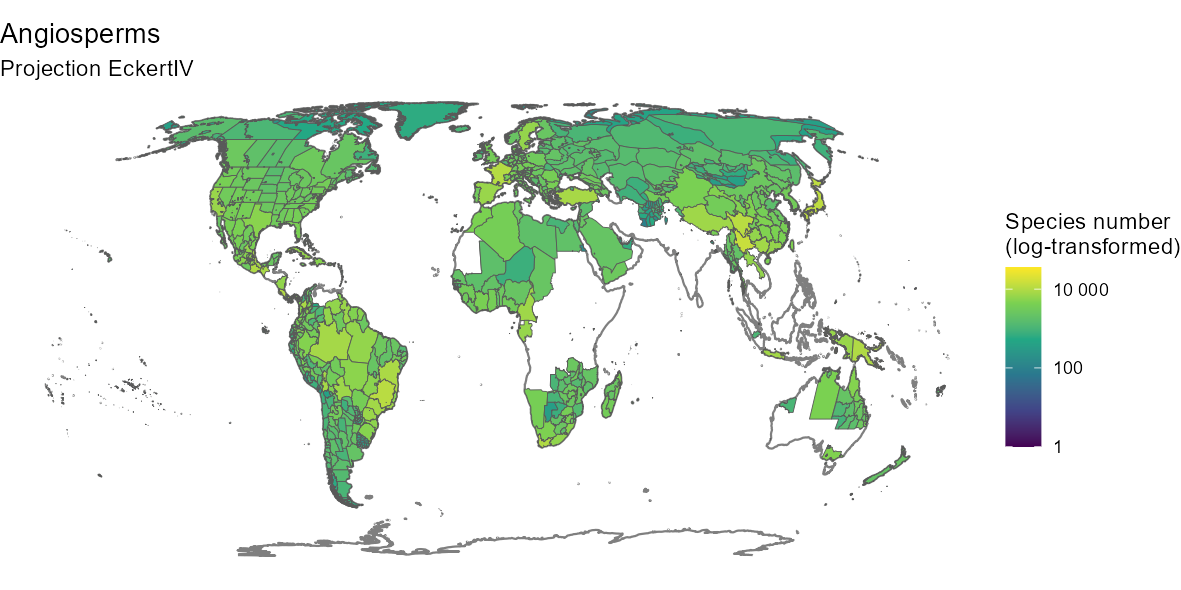By customizing the code above, you can also produce a nicer map: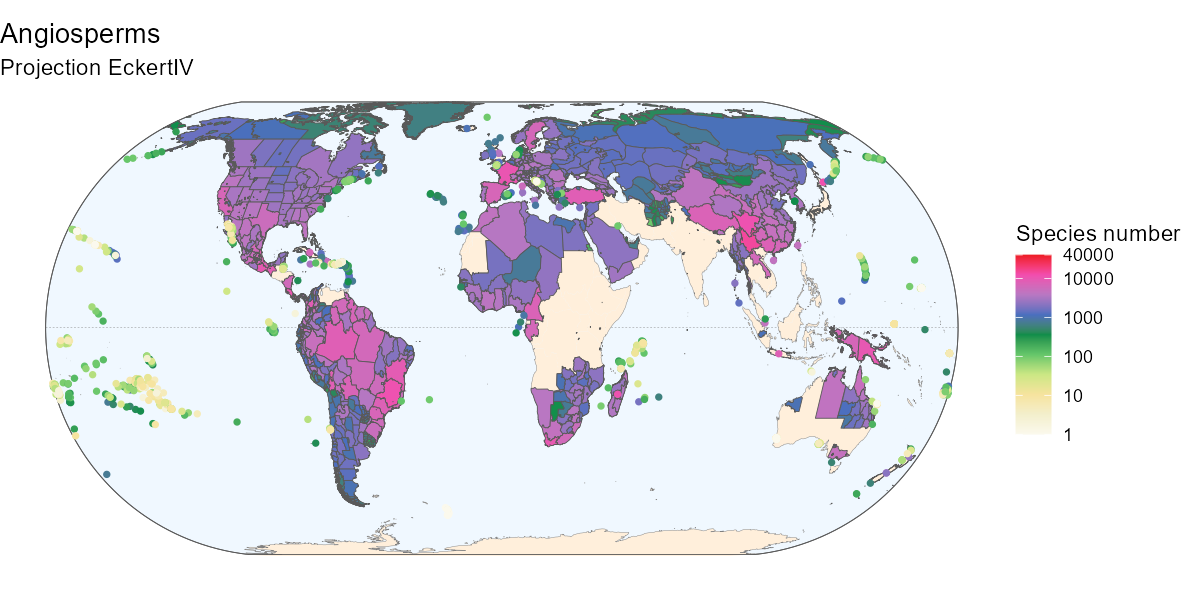Below is the R code to produce the above map if interested. Fancier code # Background box xmin <- st_bbox(world)[["xmin"]]; xmax <- st_bbox(world)[["xmax"]] ymin <- st_bbox(world)[["ymin"]]; ymax <- st_bbox(world)[["ymax"]] bb <- sf::st_union(sf::st_make_grid(st_bbox(c(xmin = xmin, xmax = xmax, ymax = ymax, ymin = ymin), crs = st_crs(4326)), n = 100)) # Equator line equator <- st_linestring(matrix(c(-180, 0, 180, 0), ncol = 2, byrow = TRUE)) equator <- st_sfc(equator, crs = st_crs(world)) # Color code from Barthlott 2007 hexcode_barthlott2007 <- c("#fbf9ed", "#f3efcc", "#f6e39e", "#cbe784", "#65c66a", "#0e8d4a", "#4a6fbf", "#b877c2", "#f24dae", "#ed1c24") ggplot(world) + geom_sf(data = bb, fill = "aliceblue") + geom_sf(data = equator, color = "gray50", linetype = "dashed", linewidth = 0.1) + geom_sf(data = world_countries, fill = "antiquewhite1", color = NA) + geom_sf(color = "gray50", linewidth = 0.1) + geom_sf(data = bb, fill = NA) + geom_sf(data = rich_map, aes(fill = ifelse(rich_map$entity_class %in%
c("Island/Mainland", "Mainland",
"Island Group", "Island Part"),
total + 1, NA)),
size = 0.1) +
geom_point(data = rich_map,
aes(color = ifelse(rich_map$entity_class %in% c("Island"), total + 1, NA), geometry = geometry), stat = "sf_coordinates", size = 1, stroke = 0.5) + scale_color_gradientn( "Species number", trans = "log10", limits = c(1, 40000), colours = hexcode_barthlott2007, breaks = c(1, 10, 100, 1000, 10000, 40000), labels = c(1, 10, 100, 1000, 10000, 40000), na.value = "transparent") + scale_fill_gradientn( "Species number", trans = "log10", limits = c(1, 40000), colours = hexcode_barthlott2007, breaks = c(1, 10, 100, 1000, 10000, 40000), labels = c(1, 10, 100, 1000, 10000, 40000), na.value = "transparent") + labs(title = "Angiosperms", subtitle = "Projection EckertIV") + coord_sf(crs = eckertIV) + theme_void() We can also produce maps of richness at intermediate scales. Here is the code and the map of Angiosperms in the Western Mediterranean basin. med_shape <- gift_shapes[which(gift_shapes$entity_ID %in%
unique(medit[]$entity_ID)), ] med_rich <- angio_rich[which(angio_rich$entity_ID %in%
unique(medit[]$entity_ID)), ] med_map <- dplyr::left_join(med_shape, med_rich, by = "entity_ID") %>% dplyr::filter(stats::complete.cases(total)) ggplot(world) + geom_sf(color = "gray50") + geom_sf(data = western_mediterranean, fill = "darkblue", color = "black", alpha = 0.1, size = 1) + geom_sf(data = med_map, aes(fill = total)) + scale_fill_viridis_c("Species number") + labs(title = "Angiosperms in the Western Mediterranean basin") + lims(x = c(-20, 20), y = c(24, 48)) + theme_void()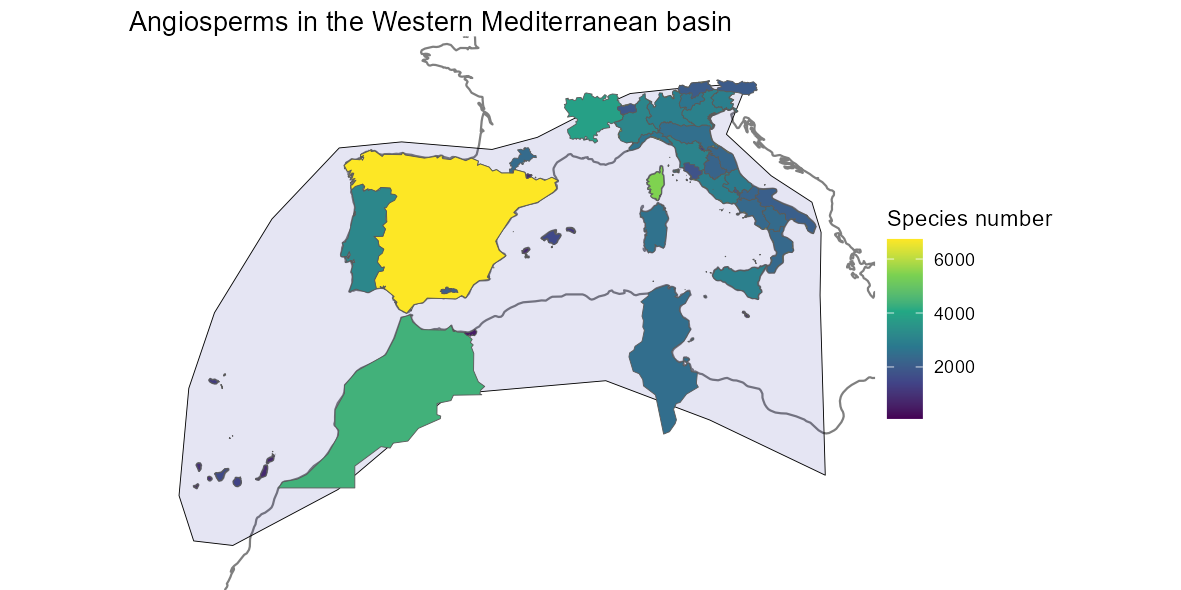## 2. Distribution of species The GIFT R package also allows for retrieving the spatial distribution of a focal plant species. ### 2.1. Available species To know what plant species are available, you can first run the function GIFT_species(). all_sp <- GIFT_species() 364571 species are currently available in the database. This number may increase with new releases of the database. See the dedicated section in the advanced vignettes for more details. ### 2.2. Species names and taxonomic harmonization Since GIFT is a collection of checklists in which authors use their own taxonomic knowledge to describe species, there is a step of taxonomic harmonization when including checklists in the database. The most commonly used backbone is the World Checklists of Vascular Plants (WCVP). Both original and harmonized names are stored in the database and you can use the GIFT_species_lookup() function to look up the differences for particular species. For example, the wood anemone Anemone nemorosa. anemone_lookup <- GIFT_species_lookup(genus = "Anemone", epithet = "nemorosa") kable(anemone_lookup, "html") %>% kable_styling(full_width = FALSE) name_ID genus species_epithet subtaxon author matched epithetscore overallscore resolved synonym matched_subtaxon accepted service work_ID taxon_ID work_genus work_species_epithet work_species work_author 3718 Anemone nemorosa NA NA 1 1 1 1 0 0 1 TNRS_wfo 2293 1303 Anemone nemorosa Anemone nemorosa L. Looking at the output table, you can see the original species names and their identification numbers (name_ID) before taxonomic harmonization. The species names and IDs after taxonomic harmonization are the last columns on the right starting with the prefix work_. ### 2.3. Species distribution Now that we have a focal species and its harmonized name, we can retrieve its distribution using GIFT_species_distribution(). Note that here we set the aggregation argument to TRUE in order to have only one floristic status per polygon. See the function’s help page for more details. anemone_distr <- GIFT_species_distribution( genus = "Anemone", epithet = "nemorosa", aggregation = TRUE) anemone_statuses <- anemone_distr %>% mutate(native = ifelse(native == 1, "native", "non-native"), naturalized = ifelse(naturalized == 1, "naturalized", "non-naturalized"), endemic_list = ifelse(endemic_list == 1, "endemic_list", "non-endemic_list")) %>% dplyr::select(entity_ID, native, naturalized, endemic_list) table(anemone_statuses$endemic_list)
##
## non-endemic_list
##                7

This species is not listed as endemic in any of the GIFT polygons. Let’s check the places where it is listed as native or naturalized.

table(paste(anemone_statuses$native, anemone_statuses$naturalized,
sep = "_"))
##
##                  NA_NA              native_NA native_non-naturalized
##                      7                      6                     16
##          non-native_NA non-native_naturalized
##                      3                      1

Looking at the different combinations of statuses, we can distinguish several situations: in 13 polygons, there is no status available. The species is listed as native and non-naturalized (or naturalized status is missing) in 113+17=130 polygons. It is naturalized and non native in 5 polygons.
More surprising are the cases where the species is non-native and non-naturalized, which happens in 3+2=5 polygons. This particular combination can occur in unstable cases where the species is in the process of becoming naturalized.

Now that we know in which polygons the species occurs and with what status, we can map its distribution using the GIFT shapes we retrieved earlier with GIFT_shapes().

# We rename the statuses based on the distinct combinations
anemone_statuses <- anemone_statuses %>%
mutate(Status = case_when(
native == "native" & naturalized == "non-naturalized" ~ "native",
native == "native" & is.na(naturalized) ~ "native",
native == "non-native" & is.na(naturalized) ~ "non-native",
native == "non-native" & naturalized == "naturalized" ~ "naturalized",
native == "non-native" & naturalized == "non-naturalized" ~ "non-native",
is.na(native) & is.na(naturalized) ~ "unknown"
))

# Merge with the shapes
anemone_shape <- gift_shapes[which(gift_shapes$entity_ID %in% unique(anemone_distr$entity_ID)), ]
anemone_map <- dplyr::left_join(anemone_shape, anemone_statuses,
by = "entity_ID")

# Area of distribution with floristic status
ggplot(world) +
geom_sf(color = "gray70") +
geom_sf(data = anemone_map, color = "black", aes(fill = as.factor(Status))) +
scale_fill_brewer("Status", palette = "Set2") +
labs(title = expression(paste("Distribution map of ",
italic("Anemone nemorosa"))),
subtitle = "Unprojected (GCS: WGS84)") +
lims(x = c(-65, 170), y = c(-45, 70)) +
theme_void()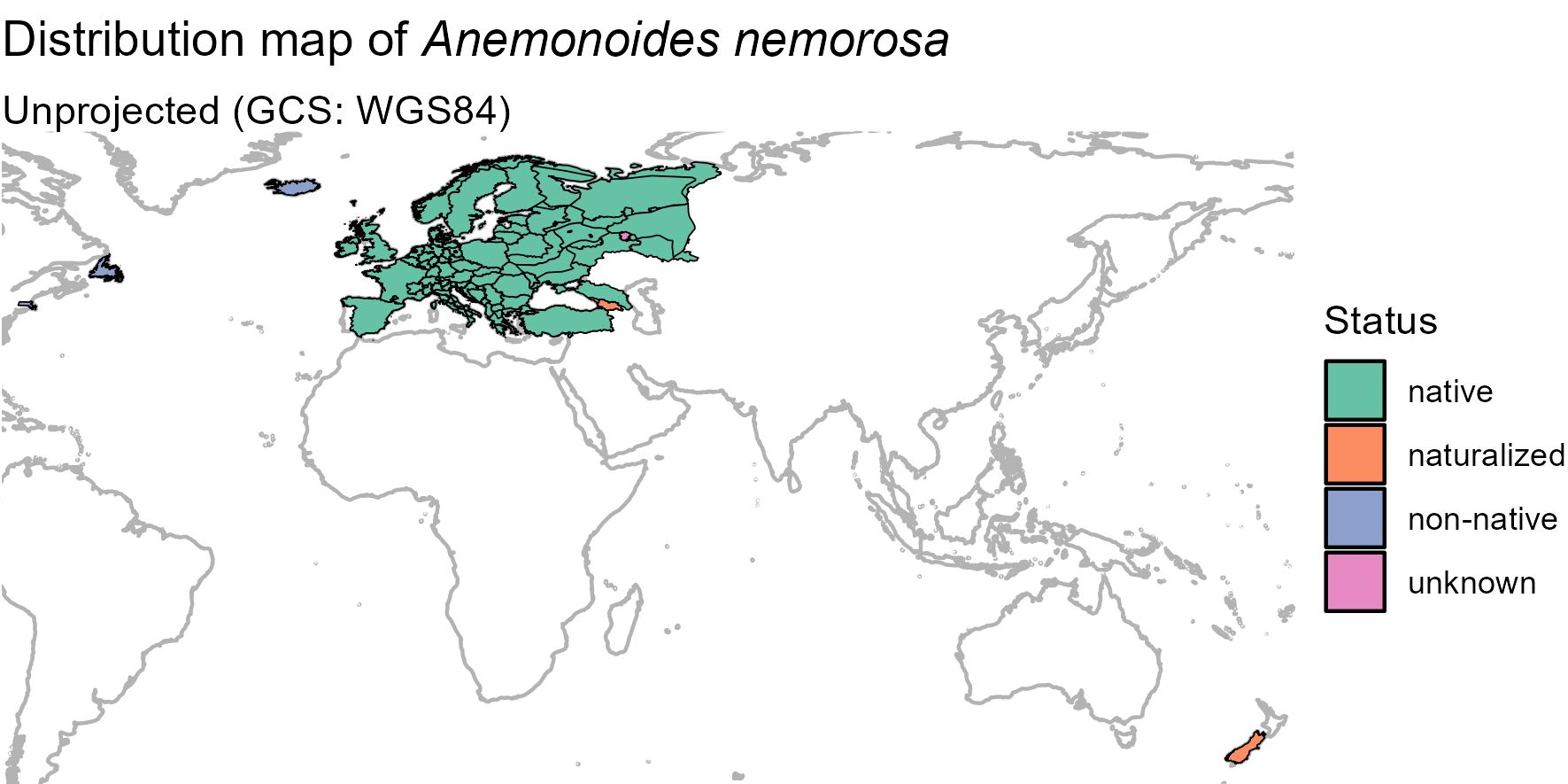By customizing the code above, you can also produce a nicer map: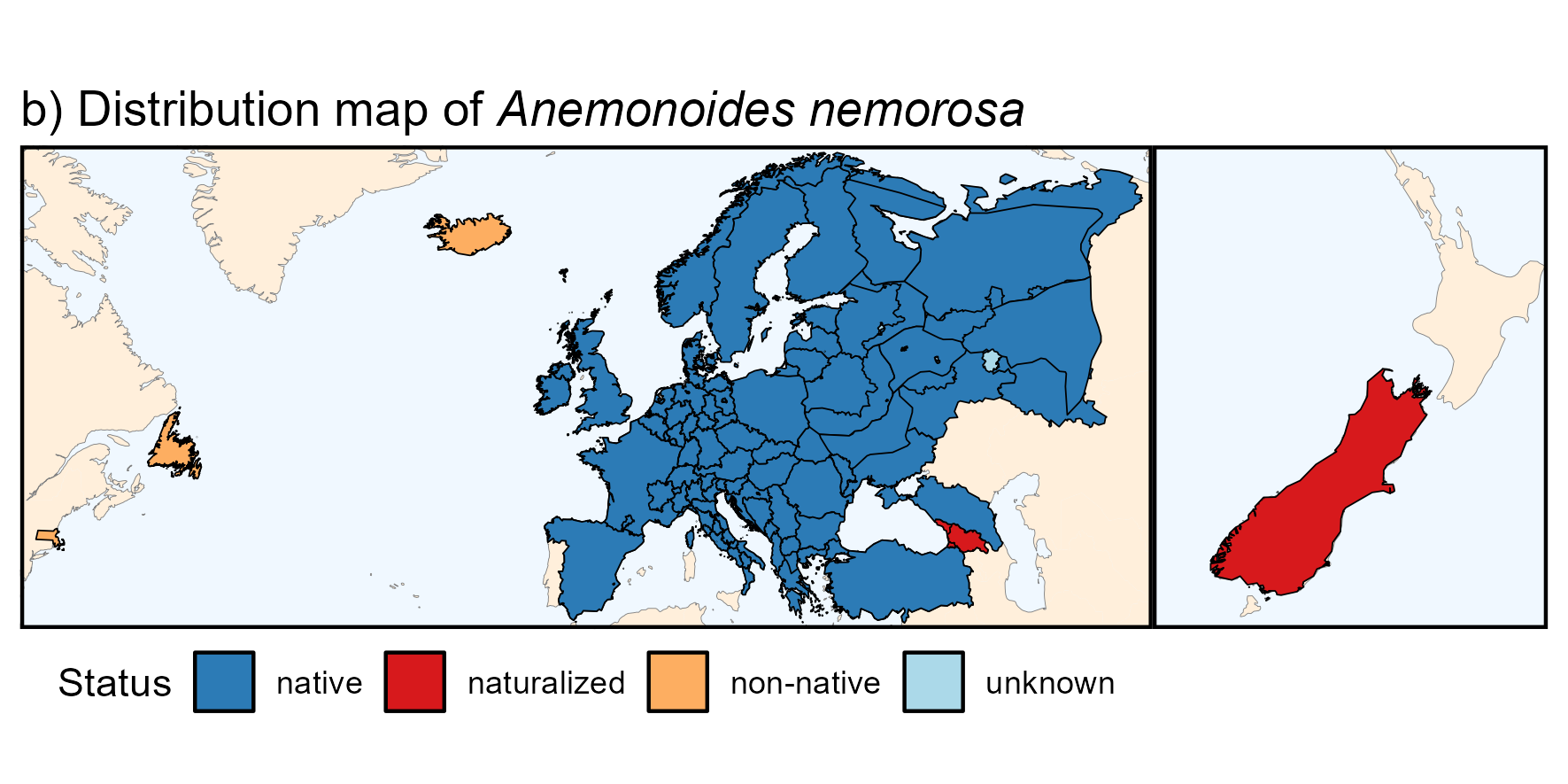Below is the R code to produce the above map if interested.

Fancier code
anemone_map_plot_bg_parts <-
ggplot(world) +
geom_sf(data = bb, fill = "aliceblue", color = NA) +
geom_sf(data = equator, color = "gray50", linetype = "dashed",
linewidth = 0.1) +
geom_sf(data = world_countries, fill = "antiquewhite1", color = NA) +
geom_sf(color = "gray50", linewidth = 0.1) +
geom_sf(data = bb, fill = NA) +
geom_sf(data = anemone_map, color = "black", aes(fill = as.factor(Status))) +
scale_fill_manual("Status",
values = c("native" = "#2c7bb6",
"naturalized" = "#d7191c",
"non-native" = "#fdae61",
"unknown" = "#abd9e9")) +
labs(title = expression(paste("b) Distribution map of ",
italic("Anemone nemorosa")))) +
theme_void() +
theme(axis.title = element_blank(),
axis.text = element_blank(),
axis.ticks = element_blank())

(anemone_map_plot_bg_parts +
lims(x = c(-69, 61), y = c(37, 70)) + # Europe & Newfoundland
theme(panel.border = element_rect(fill = NA, linewidth = 1)) +
theme(legend.position = "bottom")
|
anemone_map_plot_bg_parts +
lims(x = c(165, 178), y = c(-47, -35)) + # new zealand
labs(title = "") +
guides(fill = "none") +
theme(panel.border = element_rect(fill = NA, linewidth = 1)))

## 3. Trait data

Trait information at the species or at a higher taxonomic level is also provided in the GIFT R package.

There are many functional traits available in GIFT. Each of these traits has an identification number called trait_ID. Since the two functions for retrieving trait values, GIFT_traits() and GIFT_traits_raw(), rely on these IDs, the first step is to call the function GIFT_traits_meta() to know what the ID of the desired trait is.
For example, let’s say we want to retrieve the maximum vegetative heights of plant species.

trait_meta <- GIFT_traits_meta()
trait_meta[which(trait_meta$Trait2 == "Plant_height_max"), ] ## Lvl1 Category Lvl2 Trait1 Lvl3 Trait2 Units type ## 12 1 Morphology 1.6 Plant height 1.6.2 Plant_height_max m numeric ## comment count ## 12 <NA> 70231 We can see that the ID of this trait is 1.6.2. Now that we have the ID, we can use GIFT_traits() to retrieve the growth form values for different plant species. ### 3.2. Trait values #### 3.2.1. Species level There are two functions to access trait values. First, GIFT_traits_raw() returns all trait values for a given species and a given trait. These trait values can then vary. Second, GIFT_traits() returns an aggregated trait value at the species level. The aggregation simply takes the mean for continuous traits or the most frequent entry for categorical traits. However, for some specific cases, the aggregation takes either the minimum or the maximum, as for the trait we chose. Let’s retrieve the raw and aggregated values for the maximum vegetative height of plants (trait_ID 1.6.2). height <- GIFT_traits(trait_IDs = c("1.6.2"), agreement = 0.66, bias_ref = FALSE, bias_deriv = FALSE) height_raw <- GIFT_traits_raw(trait_IDs = c("1.6.2")) # Raw values as.numeric(height_raw[which(height_raw$work_species == "Fagus sylvatica"),
"trait_value"])

# Aggregated value
as.numeric(height[which(height$work_species == "Fagus sylvatica"), "trait_value_1.6.2"]) There were three maximum heights for Fagus sylvatica, 30, 35, and 50 meters, which led to an aggregated value of 50 meters. And if you want to look up the references that led to the aggregated trait value, you can run this chunk: references <- GIFT_references(GIFT_version = "beta") unique(unlist(strsplit(height$references_1.6.2, ",")))

references <- references[
which(references$ref_ID %in% unique(unlist(strsplit(height$references_1.6.2, ",")))), ]
references[1:2, ]

#### 3.2.2. Taxonomic level

Traits can also be retrieved at a higher taxonomic level, using GIFT_traits_tax().
As an example, we here attempt to retrieve three traits at the family level. The three traits asked are woodiness (trait_ID = "1.1.1"), growth form (trait_ID = "1.2.1") and whether the plant is a climber (trait_ID = "1.4.1").

trait_tax <- GIFT_traits_tax(trait_IDs = c("1.1.1", "1.2.1", "1.4.1"),
bias_ref = FALSE, bias_deriv = FALSE)

trait_tax[1:3, ]

Of the three requested traits, the growth form was not available at the family level. Therefore, the output table contains trait values for the other two traits at the family level.

### 3.3. Trait coverage

We can also retrieve trait coverage information for polygons, using the same function as for species richness GIFT_coverage().
In combination with the previously loaded shapes, we can also map the trait coverage.

angio_height <- GIFT_coverage(what = "trait_coverage",
taxon_name = "Angiospermae",
trait_ID = "1.6.2")

angio_height_shape <- gift_shapes[which(gift_shapes$entity_ID %in% unique(angio_height$entity_ID)), ]

angio_height_map <- dplyr::left_join(
angio_height_shape, angio_height, by = "entity_ID")

angio_height_map <- angio_height_map[complete.cases(angio_height_map$native), ] ggplot(world) + geom_sf(color = "gray50") + geom_sf(data = angio_height_map[complete.cases(angio_height_map$native), ],
aes(fill = native)) +
scale_fill_viridis_c("Coverage (%)") +
labs(title = "Coverage for maximal vegetative height of Angiosperms",
subtitle = "Projection EckertIV") +
coord_sf(crs = eckertIV) +
theme_void()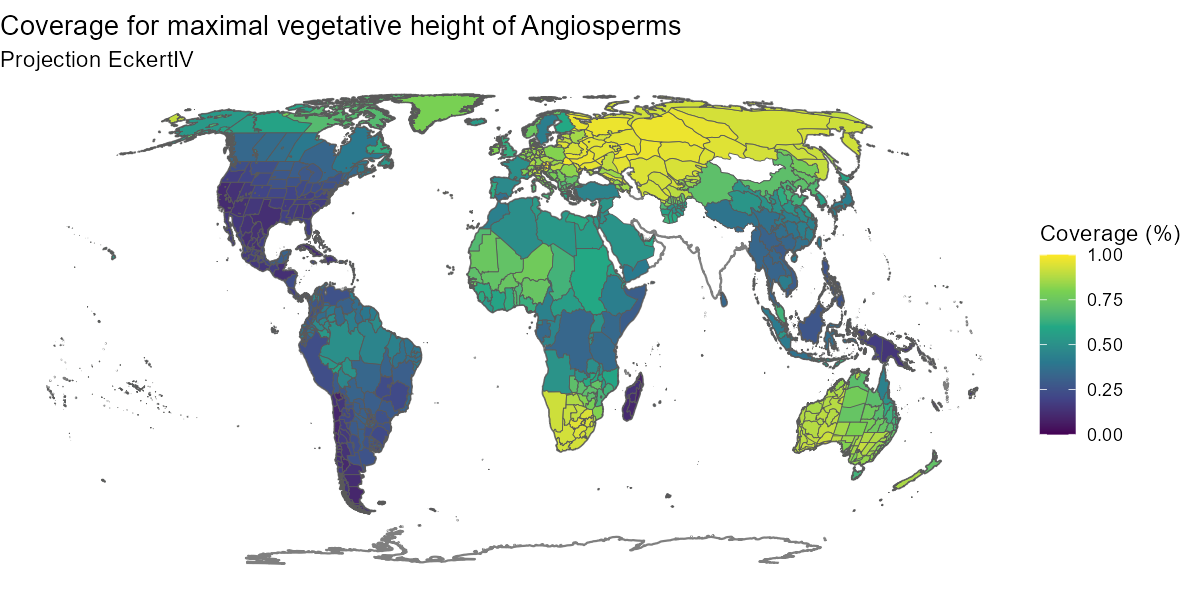By customizing the code above, you can also produce a nicer map: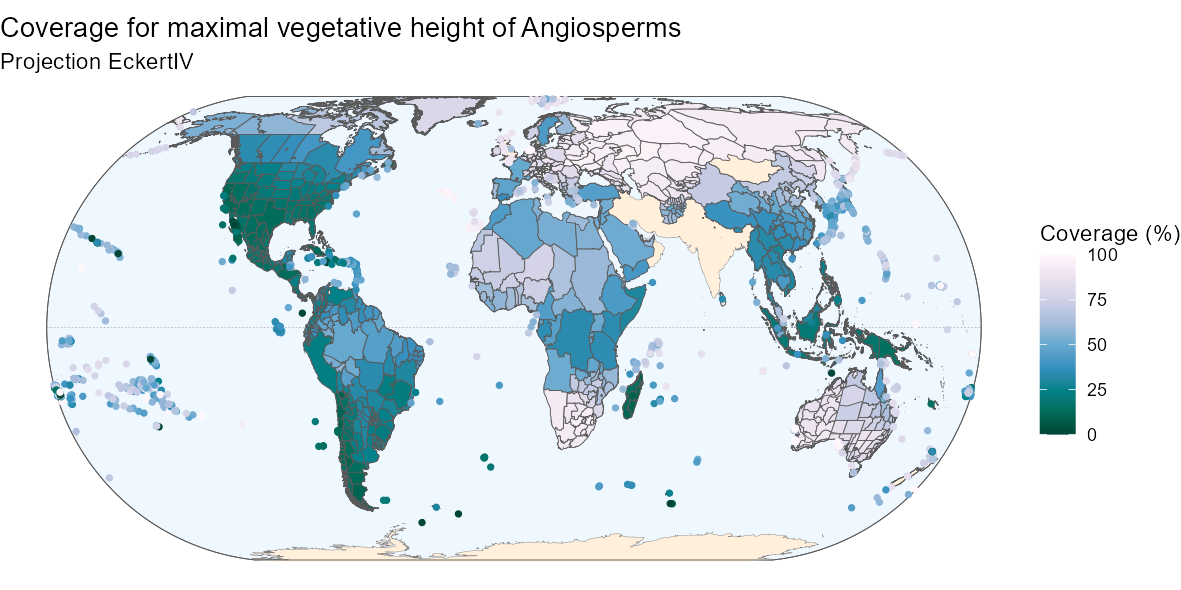Below is the R code to produce the above map if interested.

Fancier code
ggplot(world) +
geom_sf(data = bb, fill = "aliceblue") +
geom_sf(data = equator, color = "gray50", linetype = "dashed",
linewidth = 0.1) +
geom_sf(data = world_countries, fill = "antiquewhite1", color = NA) +
geom_sf(color = "gray50", linewidth = 0.1) +
geom_sf(data = bb, fill = NA) +
geom_sf(data = angio_height_map,
aes(fill = ifelse(angio_height_map$entity_class %in% c("Island/Mainland", "Mainland", "Island Group", "Island Part"), 100*native, NA)), size = 0.1) + geom_point(data = angio_height_map, aes(color = ifelse(angio_height_map$entity_class %in%
c("Island"),
100*native, NA),
geometry = geometry),
stat = "sf_coordinates", size = 1, stroke = 0.5) +
"Coverage (%)",
colours = rev(RColorBrewer::brewer.pal(9, name = "PuBuGn")),
limits = c(0, 100),
na.value = "transparent") +
"Coverage (%)",
colours = rev(RColorBrewer::brewer.pal(9, name = "PuBuGn")),
limits = c(0, 100),
na.value = "transparent") +
labs(title = "Coverage for maximal vegetative height of Angiosperms",
subtitle = "Projection EckertIV") +
coord_sf(crs = eckertIV) +
theme_void()

## 4. Environmental variables

Finally, a set of summary statistics for many environmental variables can be retrieved for each GIFT polygon.

We here illustrate how to retrieve environmental variables, summarized at the polygon level, for a subset of polygons.
We here retrieve environmental variables for the polygons falling into the western Mediterranean basin, retrieved in the section 1.
To know what variables are available, you can run these two metadata functions: GIFT_env_meta_misc() and GIFT_env_meta_raster(). They respectively give access to the list of miscellaneous variables and raster layers available in the GIFT database.
The references to cite when using environmental variables are also available through these functions (column ref_long of the outputs).

misc_env <- GIFT_env_meta_misc()
raster_env <- GIFT_env_meta_raster()

### 4.2. Environmental values

Let’s say we want to retrieve the perimeter and biome of each polygon as well as elevation and mean temperature. For these two raster layers, we need to define summary statistics, since the polygons are usually larger than the raster resolution.
There are many summary statistics available, you can check the help page of GIFT_env() to see them all. Let’s get the mean and median of the elevation and the maximal value of the average temperature.

med_env <- GIFT_env(entity_ID = unique(medit[]$entity_ID), miscellaneous = c("perimeter", "biome"), rasterlayer = c("mn30_grd", "wc2.0_bio_30s_01"), sumstat = list(c("mean", "med"), "max")) med_env[1, ] We see here that the region of El Hierro has an average altitude of 579 meters above sea level and an average annual temperature of 21.6 degrees Celsius. ### 4.3. Map Using the previously loaded shapes, we can also map a specific environmental variable for all GIFT polygons. world_temp <- GIFT_env(entity_ID = unique(angio_rich$entity_ID),
rasterlayer = c("wc2.0_bio_30s_01"),
sumstat = c("mean"))

temp_shape <- gift_shapes[which(gift_shapes$entity_ID %in% unique(angio_rich$entity_ID)), ]

temp_map <- dplyr::left_join(temp_shape, world_temp, by = "entity_ID")

ggplot(world) +
geom_sf(color = "gray50") +
geom_sf(data = temp_map, aes(fill = mean_wc2.0_bio_30s_01)) +
scale_fill_viridis_c("Celsius degrees") +
labs(title = "Average temperature",
subtitle = "Projection EckertIV") +
coord_sf(crs = eckertIV) +
theme_void()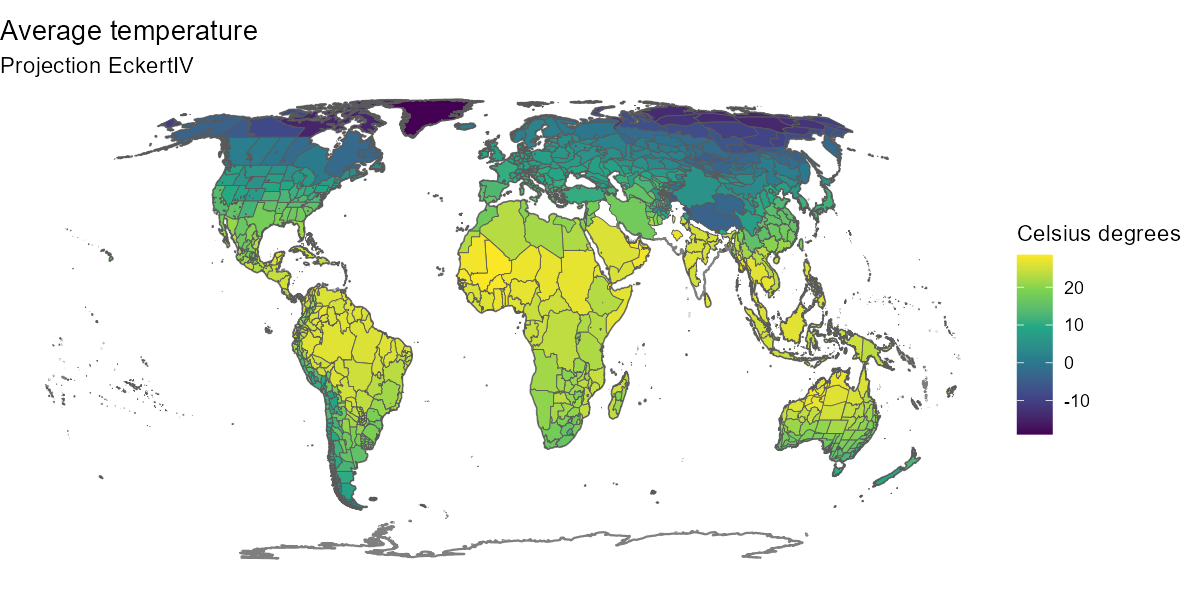By customizing the code above, you can also produce a nicer map: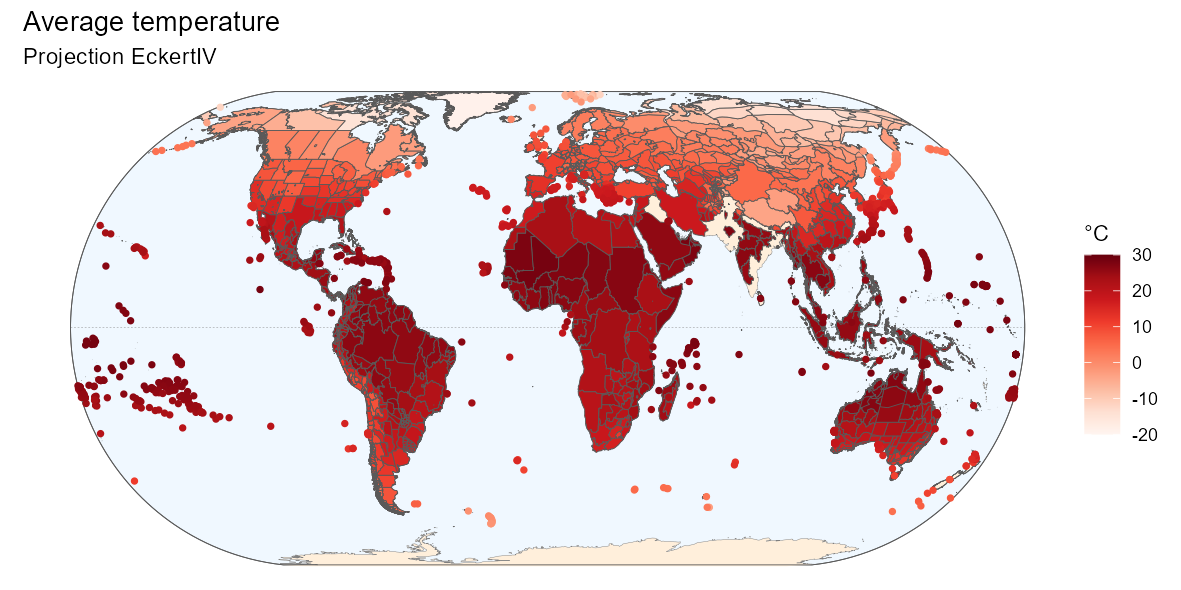Below is the R code to produce the above map if interested.

Fancier code
ggplot(world) +
geom_sf(data = bb, fill = "aliceblue") +
geom_sf(data = equator, color = "gray50", linetype = "dashed",
linewidth = 0.1) +
geom_sf(data = world_countries, fill = "antiquewhite1", color = NA) +
geom_sf(color = "gray50", linewidth = 0.1) +
geom_sf(data = bb, fill = NA) +
geom_sf(data = temp_map,
aes(fill = ifelse(temp_map$entity_class %in% c("Island/Mainland", "Mainland", "Island Group", "Island Part"), mean_wc2.0_bio_30s_01, NA)), size = 0.1) + geom_point(data = temp_map, aes(color = ifelse(temp_map$entity_class %in%
c("Island"),
mean_wc2.0_bio_30s_01, NA),
geometry = geometry),
stat = "sf_coordinates", size = 1, stroke = 0.5) +
"°C",
colours = RColorBrewer::brewer.pal(9, name = "Reds"),
limits = c(-20, 30),
na.value = "transparent") +
"°C",
colours = RColorBrewer::brewer.pal(9, name = "Reds"),
limits = c(-20, 30),
na.value = "transparent") +
labs(title = "Average temperature",
subtitle = "Projection EckertIV") +
coord_sf(crs = eckertIV) +
theme_void()

## 5. Phylogeny

To build a phylogeny for all species in the GIFT database, we have used the U.PhyloMaker R package Jin & Qian (2022). For seed plants, we selected the megatree GBOTB.extended.WP.tre by Smith and Brown (2018) and the phylogeny for pteridophytes in Zanne et al. (2014).

# Retrieving phylogeny, taxonomy and species from GIFT

gf <- GIFT_traits(trait_IDs = "1.2.1", agreement = 0.66, bias_ref = FALSE,
bias_deriv = FALSE, GIFT_version = "beta")
# Replacing space with _ for the species names
gf$work_species <- gsub(" ", "_", gf$work_species, fixed = TRUE)
# Retrieving family of each species
sp_fam <- GIFT_taxgroup(work_ID = unique(gift_sp$work_ID), taxon_lvl = "family", GIFT_version = "beta") sp_genus_fam <- data.frame( work_ID = unique(gift_sp$work_ID),
work_species = unique(gift_sp$work_species), family = sp_fam) sp_genus_fam <- left_join(sp_genus_fam, gift_sp[, c("work_ID", "work_genus")], by = "work_ID") colnames(sp_genus_fam)[colnames(sp_genus_fam) == "work_genus"] <- "genus" # Problem with hybrid species on the tip labels of the phylo tree phy$tip.label[substring(phy$tip.label, 1, 2) == "x_"] <- substring(phy$tip.label[substring(phy$tip.label, 1, 2) == "x_"], 3, nchar(phy$tip.label[substring(phy$tip.label, 1, 2) == "×_"])) phy$tip.label[substring(phy$tip.label, 1, 2) == "×_"] <- substring(phy$tip.label[substring(phy$tip.label, 1, 2) == "×_"], 3, nchar(phy$tip.label[substring(phy$tip.label, 1, 2) == "×_"])) In the next chunk, we calculate the trait coverage (for growth form) at the genus and family level. sp_genus_fam <- left_join(sp_genus_fam, gf[, c("work_ID", "trait_value_1.2.1")], by = "work_ID") genus_gf <- sp_genus_fam %>% group_by(genus) %>% mutate(prop_gf = round(100*sum(is.na(trait_value_1.2.1))/n(), 2)) %>% ungroup() %>% dplyr::select(-work_ID, -work_species, -family, -trait_value_1.2.1) %>% distinct(.keep_all = TRUE) fam_gf <- sp_genus_fam %>% group_by(family) %>% mutate(prop_gf = round(100*sum(is.na(trait_value_1.2.1))/n(), 2)) %>% ungroup() %>% dplyr::select(-work_ID, -work_species, -genus, -trait_value_1.2.1) %>% distinct(.keep_all = TRUE) sp_genus_fam$species <- gsub("([[:punct:]])|\\s+", "_",
sp_genus_fam$work_species) # Keeping one species per genus only one_sp_per_gen <- data.frame() for(i in 1:n_distinct(sp_genus_fam$genus)){ # loop over genera
# Focal genus
focal_gen <- unique(sp_genus_fam$genus)[i] # All species in that genus gen_sp_i <- sp_genus_fam[which(sp_genus_fam$genus == focal_gen),
"species"]
# Species from the genus available in the phylogeny
gen_sp_i <- gen_sp_i[gen_sp_i %in% phy$tip.label] # Taking the first one (if at least one is available) gen_sp_i <- gen_sp_i one_sp_per_gen <- rbind(one_sp_per_gen, data.frame(species = gen_sp_i, genus = focal_gen)) } # Adding the trait coverage per genus one_sp_per_gen <- left_join(one_sp_per_gen, genus_gf, by = "genus") # Adding the trait coverage per family one_sp_per_gen <- left_join(one_sp_per_gen, sp_genus_fam[!duplicated(sp_genus_fam$genus),
c("genus", "family")],
by = "genus")
colnames(one_sp_per_gen)[colnames(one_sp_per_gen) == "prop_gf"] <-
"prop_gf_gen"
one_sp_per_gen <- left_join(one_sp_per_gen, fam_gf, by = "family")
colnames(one_sp_per_gen)[colnames(one_sp_per_gen) == "prop_gf"] <-
"prop_gf_fam"

We now prune the tree at the genus level.

phy_gen <- ape::keep.tip(
phy = phy,
tip = one_sp_per_gen[complete.cases(one_sp_per_gen\$species), "species"])

In the following plot, there is only one tip per genus. The two outer rings illustrate the coverage of growth form per genus and per family (outer ring). For the family ring, the width of each family is set by the number of genera it contains.
To get the following plot, you need to install/load the following packages:

library("BiocManager")
install("ggtree")
library("ggtree")
library("tidytree")
install("ggtreeExtra")
library("ggtreeExtra")

ggtree(phy_gen, color = "grey70", layout = "circular") %<+% one_sp_per_gen +
geom_fruit(geom = geom_tile,
mapping = aes(fill = prop_gf_gen),
width = 50,
offset = 0.1) +
geom_fruit(geom = geom_tile,
mapping = aes(color = prop_gf_fam, fill = prop_gf_fam),
width = 50,
offset = 0.1,
show.legend = FALSE) +
scale_color_viridis_c() +
scale_fill_viridis_c("Growth form availability per genus (%)") +
theme(legend.position = "bottom")